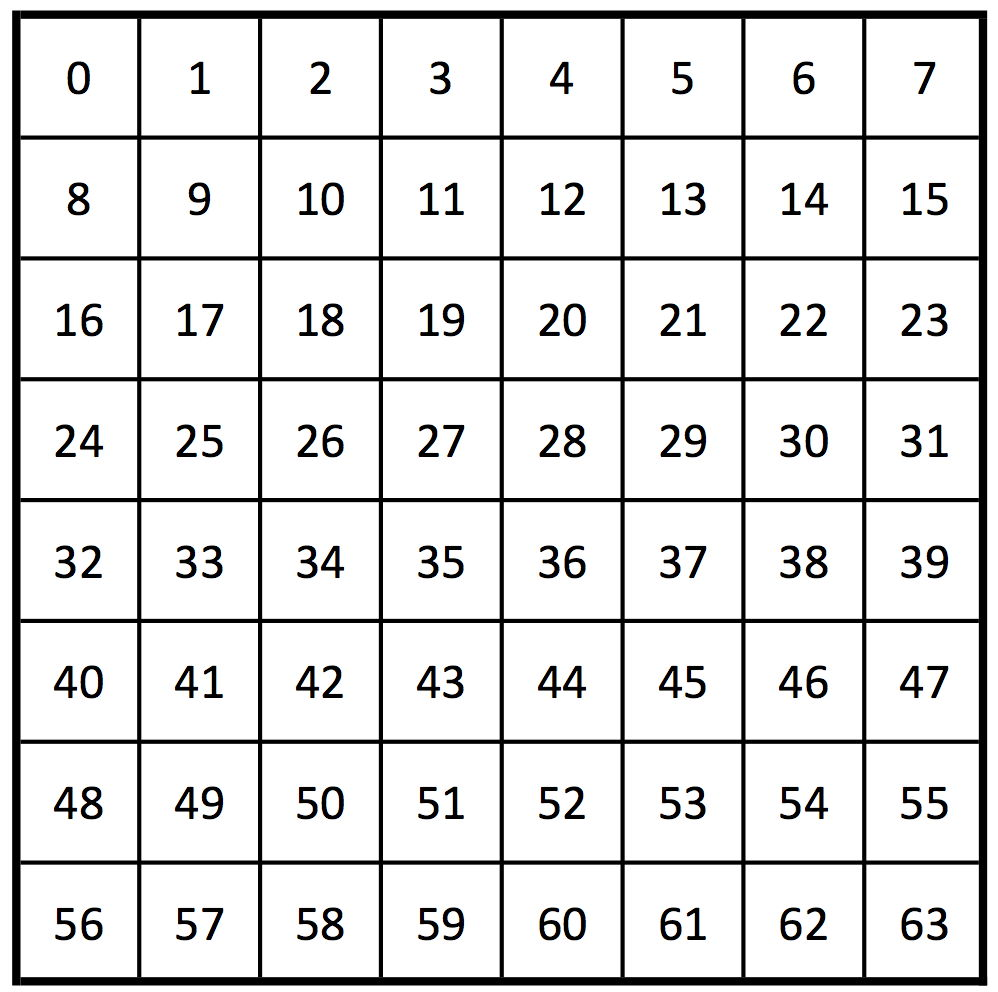시간 제한메모리 제한제출정답맞힌 사람정답 비율
1 초 512 MB57343368.750%

## 문제

Zahhak, the enemy of Jamshid, has captured Jamshid's daughters, Arnavaz and Shahrnaz. But he decided to offer them an opportunity to free themselves by solving a puzzle.

Zahhak has an $8 \times 8$ chessboard with cells labeled from $0$ to $63$, as in the figure. He has put a coin on each of the $64$ cells. The cell with label $c$ has a special coin which is physically identical to the other coins, but it is cursed. Each coin is facing either heads or tails.Zahhak invited the sisters to dinner to describe the puzzle: after the dinner, the sisters should go to different rooms. Then Zahhak goes to Arnavaz's room, presents her the chessboard and tells her the value of $c$ (the label of the cell containing the cursed coin). Arnavaz cannot change the position of the coins but can flip (turn over) at least $1$ and at most $k$ coins. She might flip the same coin several times. Then Zahhak goes to the other room and presents the chessboard to Shahrnaz. If she finds the cursed coin, both sisters will be freed. The sisters can agree on a strategy during the dinner, but cannot communicate afterward.

## 구현

You should implement two different procedures:

int[] coin_flips(int[] b, int c)

• This procedure plays for Arnavaz.
• $b$: an integer array of length $64$, demonstrating the chessboard that Zahhak presents to Arnavaz. The value of $b[i]$ (for $0 \leq i \leq 63$) is either $0$ or $1$, which indicates the coin on cell $i$ is heads or tails, respectively.
• $c$: label of the cell that contains the cursed coin.
• It should return an array containing labels of the cells that Arnavaz flips their coins. Its length should be between $1$ and $k$, inclusive. It can contain a value more than once.
int find_coin(int[] b)

• This procedure plays for Shahrnaz.
• $b$: an integer array of length $64$, demonstrating the chessboard that Zahhak presents to Shahrnaz (after Arnavaz has flipped some coins).
• It should return $c$, the position of the cursed coin.

There are $T$ scenarios. For each scenario, the grader calls the procedure coin_flips. Based on its returned value, the grader updates the chessboard and calls the procedure find_coin. Note that in the judging system these procedures are called in separate programs. In the first program, procedure coin_flips is called once for each scenario. Invocations of procedure find_coin are made in the second program. The behavior of your implementation for each scenario must be independent of the order of the scenarios, as scenarios might not have the same order in the two programs.

## 제한

• $1 \leq T \leq 1000$,
• $0 \leq c \leq 63$.

## 예제

Suppose the sisters decide that Arnavaz only flips the cursed coin and Shahrnaz reports the position of one of the coins with tails facing up, or $0$ if there is no such coin. Clearly, this is just an example, not a correct strategy.

The grader makes the following procedure call:

coin_flips([0,0,...,0,0], 63)


In this example, $b$ is an array of length $64$ filled with $0$'s which means all coins on the chessboard have heads facing up. This procedure returns an integer array of length $1$, containing a single value $$.

Then the grader flips the coin in the cell number $63$ and makes the following procedure call:

find_coin([0,0,...,0,1])


This procedure returns $63$, and it is the correct position of the cursed coin.

## 서브태스크

번호 배점 제한
1 10

$c < 2$, $k = 1$

2 15

$c < 3$, $k = 1$

3 10

$k = 64$

4 15

$k = 8$

5 50

$k = 1$

## 샘플 그레이더

• line $1$: $\;\;T$
• block $i$ (for $0 \leq i \leq T-1$): a block of $9$ lines, representing scenario $i$.
• line $1$: $\;\;c$
• line $2+j$ (for $0 \leq j \leq 7$): a binary (0/1) string of length $8$ representing row $j$ of table $b$

The sample grader writes the output in the following format:

• line $1+i$ (for $0 \leq i \leq T-1$): the verdict of your solution for scenario $i$.

## 제출할 수 있는 언어

C++17, C++14, C++20, C++14 (Clang), C++17 (Clang), C++20 (Clang)

## 채점 및 기타 정보

• 예제는 채점하지 않는다.